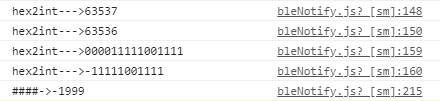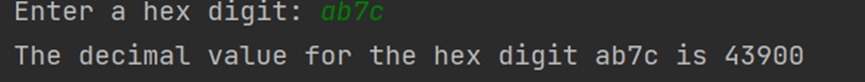• 十六进制数怎么转十进制
千次阅读
2020-12-04 18:29:13

int main()
{
unsigned int a = 0xd8;
if (a & 0x80) {
a -= 1;
a = ~a;
a &= 0x7f;
a = -a;
}
printf("a %d\n", a);
return 0;
}

示例的变量为八位数

更多相关内容
• 基于C实现二、八、十六进制数转十进制数的算法，最终封装到一个函数里面，使用方便！！！
• 　从键盘输入一个不超过8位的正的十六进制数字符串，将它转换为正的十进制数后输出。 　注：十六进制数中的10~15分别用大写的英文字母A、B、C、D、E、F表示。 样例输入 FFFF 样例输出 65535 思路：感觉自己的...
• 今天小编就为大家分享一篇关于易语言将十进制数值转换为十六进制文本的方法，小编觉得内容挺不错的，现在分享给大家，具有很好的参考价值，需要的朋友一起跟随小编来看看吧
• 16进制10进制例程,十六进制转十进制,十六进制,十进制,属于入门级教程源码,由尽在眼前写的例子
• 前些天用pb开发的时候发现PB中没有现成的进制转化的函数，于是自己写了一个，下载下去导入一下
• 今天做小程序开发，需要将对应蓝牙特征值中的数据(十六进制字符串)转化为十进制数。 var a = "F831"; console.log("####->"+parseInt(a,16)); 发现0xF831转换为十进制后，结果却是63537。但在底层开发中，数据...

## 原文地址

Javascript 16进制转有符号的10进制整数

## 前言

今天做小程序开发，需要将对应蓝牙特征值中的数据(十六进制字符串)转化为十进制数。

var a = "F831";
console.log("####->"+parseInt(a,16));


发现0xF831转换为十进制后，结果却是63537。但在底层开发中，数据类型为int16_t(有符号 2字节)。转换的完全对不上呀！

## 正文

朋友推荐一篇参考博客。下面为转载内容。

这里我们就需要温习一下数据在底层的转换，我们都知道数据在计算机里都是用二进制形式存储的。有符号的整数有两种方式存储，一种正数存储，一种负数存储。正数存储就是以真二进制的方式，最高位为0，后面每一位都表示2的幂次。而负数则麻烦的多，它采用二进制补码的形式存储。确定一个负数的二进制需要三步：

• 1、确定该数字的绝对值的二进制，因为是负数所以最高位的“0”改为“1”，其为原码。
• 2、将该绝对值，除了最高位的符号位外其他位的“0”替换成“1”,“1”替换成“0”，这就是二进制的反码。
• 3、反码加上1，确定其补码。

比如-256转换为二进制，具体步骤如下：

• 1.先将256转换为二进制数为1 0000 0000，因位数不是8的倍数，需要在不足的高位补上0，得0000 0001 0000 0000。因为-256是负数，所以最高位改为1000 0001 0000 0000。
• 2.再将除了符号位其他位的1和0对换，得1111 1110 1111 1111。
• 3.把反码加上1得1111 1111 0000 0000。

现在再把它转为16进制，就得到上面我们一开始的“FF00”了。

ps：看到一个关于负数为什么是用补码存储的有趣说法，说计算机喜欢加法，正数的二进制和负数的二进制相加得0，这样极大减少了内存占用。

## 具体分析

首先先定义一个变量：

var i = "F831";


JavaScript只提供了2-32进制转换为10的函数，和10进制转换为2-32的方法，所以我们要把一个16进制转换为2进制需要使用其10进制作为中间量。

let two = parseInt(i, 16).toString(2);


再求出变量应有的位数，在不足的位数上补“0”。

let bitNum=i.length*4;
if (two.length < bitNum) {
while (two.length < bitNum) {
two = "0" + two;
}
}


判断它的最高位是否是0，如果是，转换为10进制后原样输出。

if (two.substring(0, 1) == "0") {
two = parseInt(two, 2);
}


如果不是，按照之前提供的步骤处理一下。

else {
let two_unsign = "";
two = parseInt(two, 2) - 1;//减一
two = two.toString(2);
two_unsign = two.substring(1, bitNum);//截取除了最高位以外的位
two_unsign = two_unsign.replace(/0/g, "z");//反码
two_unsign = two_unsign.replace(/1/g, "0");
two_unsign = two_unsign.replace(/z/g, "1");
two = parseInt(-two_unsign, 2);//补上负号
}


我们封装一下。

module.exports=(i)=>{
let two = parseInt(i, 16).toString(2);
let bitNum=i.length*4;
if (two.length < bitNum) {
while (two.length < bitNum) {
two = "0" + two;
}
}

if (two.substring(0, 1) == "0") {
two = parseInt(two, 2);

return two;
} else {
let two_unsign = "";
two = parseInt(two, 2) - 1;
two = two.toString(2);
two_unsign = two.substring(1, bitNum);
two_unsign = two_unsign.replace(/0/g, "z");
two_unsign = two_unsign.replace(/1/g, "0");
two_unsign = two_unsign.replace(/z/g, "1");
two = parseInt(-two_unsign, 2);

return two;
}
}


## js定义funtion

function hex2int(hexStr) {
let twoStr = parseInt(hexStr,16).toString(2); // 将十六转十进制，再转2进制
let bitNum = hexStr.length * 4; // 1个字节 = 8bit ，0xff 一个 "f"就是4位
if(twoStr.length < bitNum){
while(twoStr.length < bitNum){
twoStr = "0" + twoStr;
}
}
if(twoStr.substring(0,1) == "0"){
// 正数
twoStr = parseInt(twoStr,2); // 二进制转十进制
return twoStr;
}else{
// 负数
let twoStr_unsign = "";

console.log("hex2int--->"+parseInt(twoStr,2));
twoStr = parseInt(twoStr,2) - 1; // 补码：(负数)反码+1，符号位不变；相对十进制来说也是 +1，但这里是负数，+1就是绝对值数据-1
console.log("hex2int--->"+twoStr);

twoStr = twoStr.toString(2);
twoStr_unsign = twoStr.substring(1,bitNum); // 舍弃首位(符号位)
// 去除首字符，将0转为1，将1转为0   反码
twoStr_unsign = twoStr_unsign.replace(/0/g, "z");
twoStr_unsign = twoStr_unsign.replace(/1/g, "0");
twoStr_unsign = twoStr_unsign.replace(/z/g, "1");

console.log("hex2int--->"+twoStr_unsign);
console.log("hex2int--->"+(-twoStr_unsign));
twoStr = parseInt(-twoStr_unsign, 2);
return twoStr;
}
}


## 测试

var a = "F831";
console.log("####->"+hex2int(a));展开全文• 十六进制数中，用字母A-F表示十进制数中的0-15。下列程序可以将用户输入的十六进制数转换为对应的十进制数，其中用户输入时不区分大小写。 import java.util.Scanner; public class HexDigit2Dec { public ...

在十六进制数中，用字母A-F表示十进制数中的0-15。下列程序可以将用户输入的十六进制数转换为对应的十进制数，其中用户输入时不区分大小写。

import java.util.Scanner;

public class HexDigit2Dec {
public static void main(String[] args){
Scanner input=new Scanner(System.in);
System.out.print("Enter a hex digit: ");
String hexDigit=input.nextLine();

int count=0;
int value = 0;
for(int i=0;i<hexDigit.length();i++){
char ch=Character.toUpperCase(hexDigit.charAt(i));//将截取出的字符转换为大写字母
if('A'<=ch&&ch<='F'){
value=ch-'A'+10;
}
else if(Character.isDigit(ch)){
value=Integer.parseInt(String.valueOf(ch));

}
else{
System.out.println(ch+" is an invalid input");
System.exit(1);
}
count=count*16+value;
}
System.out.println("The decimal value for the hex digit "+hexDigit+" is "+count);
}
}展开全文java
• 主要介绍了C#十六进制字符串转十进制int的方法,涉及C#操作数制转换的技巧,具有一定参考借鉴价值,需要的朋友可以参考下
• 有符号十六进制转十进制，自己写的，已在项目中应用，java代码，android可以直接调用，希望对大家有帮助
• 主要介绍了C++实现十六进制字符串转换为十进制整数的方法,涉及C++字符串与数制转换的相关技巧,具有一定参考借鉴价值,需要的朋友可以参考下
• 16进制与有符号10进制间的相互转换，支持16进制数转换为有符号10进制数，或将有符号10进制转换为16进制数，可选包含1、2、4、8字节。
• 十进制数转换为十六进制数Conversion of decimal number system into hexadecimal number system can be done by successively dividing an integral part by 16 till the quotient is 0 and then reading the ...

十进制数转换为十六进制数

Conversion of decimal number system into hexadecimal number system can be done by successively dividing an integral part by 16 till the quotient is 0 and then reading the remainder of all in the bottom to the top manner, where the bottom one is the MSB and the topmost is the LSB. For fractional part, we successively multiply it by 16 till we get 0 in the fractional part of the product term, the integral part of the product term recorded from top to bottom forms the respective hexadecimal number where topmost is the MSB.

可以通过将一个整数部分依次除以16直到商为0，然后从下到上依次读取所有的余数，从而将十进制数系统转换为十六进制系统 ，其中最低的是MSB，最高的是是LSB。 对于小数部分，我们将其连续乘以16，直到乘积项的小数部分得到0，从上到下记录的乘积项的整数部分形成相应的十六进制数，其中最高的是MSB。

To convert a mixed decimal number into hexadecimal, we will first convert integral and fractional parts into hexadecimal and then combine them.

要将混合的十进制数转换为十六进制 ，我们将首先将整数和小数部分转换为十六进制，然后将它们组合。

The only thing to be kept in mind is the digits in hexadecimal number system are as:

唯一要记住的是十六进制数字中的数字为：

1 , 2, 3, 4, 5, 6, 7, 8, 9, 10 = A, 11 = B, 12 = C, 13 = D, 14 = E, 15 = F

1、2、3、4、5、6、7、8、9、10 = A，11 = B，12 = C，13 = D，14 = E，15 = F

Now let's take examples to understand the conversion of decimal number to hexadecimal number.

现在，让我们以示例了解十进制数到十六进制数转换

Example 1:

范例1：

Convert (1954.785)10 to ( ? )16

将(1954.785) 10转换为(？) 16

Solution:

解：

Given decimal number (1954.785)10 is of mixed type and contains both integral (1954)10 and decimal part (0.785)10. To convert the given number into hexadecimal, we have to convert integral and fractional part individually into hexadecimal and then combine them together to get the required result.

给定的十进制数(1954.785) 10是混合类型，并且包含整数(1954) 10和十进制部分(0.785) 10 。 要将给定数字转换为十六进制，我们必须将整数和小数部分分别转换为十六进制，然后将它们组合在一起以获得所需的结果。

Integral Part

整体部分

DivisorQuotientRemainder
161954
161222 LSB
16710 = A
1607 MSB
除数
16 1954年
16 122 2 LSB
16 7 10 = A
16 0 7个MSB

The remainders read from bottom to top gives the equivalent hexadecimal number i.e., (1954)10 = (7A2)16.

从下到上读取的余数给出等效的十六进制数，即(1954) 10 =(7A2) 16

Fractional Part

小数部分

    0.785 * 16 = 12.56 = C.56     (MSB)
0.56 * 16 = 8.96
0.96 * 16 = 15.36 = F.36
0.36 * 16 = 5.76
0.76 * 16 = 12.16 = C.16    (LSB)



The integer part of the product term read from top to bottom forms the equivalent hexadecimal number i.e., (0.785)10 = (0.C8F5C)16.

从上到下读取的乘积项的整数部分形成等效的十六进制数，即(0.785) 10 =(0.C8F5C) 16

After converting both integral part and fractional part individually into hexadecimal, now we combine both to get our desired result i.e., (1954.785)10 = (7A2.C8F5C)16.

将整数部分和小数部分分别转换为十六进制后，现在我们将两者结合起来即可得到所需的结果，即(1954.785) 10 =(7A2.C8F5C) 16

Example 2:

范例2：

Convert (3283.715)10 to ( ? )16

将(3283.715) 10转换为(？) 16

Solution:

解：

Integral Part

整体部分

DivisorQuotientRemainder
163283
162053 LSB
161213=D
16012=C MSB
除数
16 3283
16 205 3 LSB
16 12 13 = D
16 0 12 = C高位

The remainders read from bottom to top gives the equivalent hexadecimal number i.e., (3283)10 = (CD3)16.

从下到上读取的余数给出等效的十六进制数，即(3283) 10 =(CD3) 16

Fractional Part

小数部分

    0.715 * 16 = 11.44 = B.44   (MSB)
0.44 * 16 = 7.04
0.04 * 16 = 0.64
0.64 * 16 = 10.24 = A.24    (LSB)



The integer part of the product term read from top to bottom forms the equivalent hexadecimal number i.e., (0.715)10 = (0. B70A)16.

从上到下读取的乘积项的整数部分形成等效的十六进制数，即(0.715) 10 =(0. B70A) 16

After converting both integral part and fractional part individually into hexadecimal, now we combine both to get our desired result i.e., (3283.715)10 = (CD3. B70A)16.

在将整数部分和小数部分分别转换为十六进制之后，现在我们将两者合并以获得所需的结果，即(3283.715) 10 =(CD3。B70A) 16

Example 3:

范例3：

Convert (356.225)10 to ( ? )16

将(356.225) 10转换为(？) 16

Solution:

解：

Integral Part

整体部分

DivisorQuotientRemainder
16356
16224 LSB
1616
1601 MSB
除数
16 356
16 22 4 LSB
16 1个 6
16 0 1个MSB

The remainders read from bottom to top gives the equivalent hexadecimal number i.e., (356)10 = (164)16.

从下到上读取的余数给出等效的十六进制数，即(356) 10 =(164) 16

Fractional Part

小数部分

    0.225 * 16 = 3.600		(MSB)
0.600 * 16 = 9.600
0.600 * 16 = 9.600
0.600 * 16 = 9.600		(LSB)



The integer part of the product term read from top to bottom forms the equivalent hexadecimal number i.e., (0.225)10 = (0.39)16.

从上到下读取的乘积项的整数部分形成等效的十六进制数，即(0.225) 10 =(0.3 9 ) 16

After converting both integral part and fractional part individually into hexadecimal, now we combine both to get our desired result i.e., (356.225)10 = (164.39)16.

在将整数部分和小数部分分别转换为十六进制之后，现在我们将两者结合以获得所需的结果，即(356.225) 10 =(164.3 9 ) 16

十进制数转换为十六进制数

展开全文clang java
• 将键盘输入的十六进制数转换为十进制输出。 实验原理 首先利用dos功能调用，读取输入的两位十六进制的第一位数字，首先判断输入的字符是否在0-9、a-f、A-F之间，如果不在则程序直接退出，如果在则将大写字母减37h，...
• labview十六进制转十进制，输入字符串控件是十六进制显示。
• 最近由于工作上的需要，遇到进制转换的问题。涉及到的进制主要是 十进制十六进制，二进制中间的转换。还包括了一个字节数组 与十六进制字符串的互相转换。估计遇到蓝牙通信的朋友，应该用的上。
• 十六进制转换十进制原理介绍 十六进制转换为十进制，是先要将十六进制...十六进制F对应二进制1111，十六进制6对应二进制0110，十六进制C对应二进制1100，那么十六进制数0x1F6C对应的二进制数就是0001 1111 0110 1100。
• 十六进制字符串转换为十进制数，一个小技巧，仅供参考学习
• 十进制转换为二进制、八进制、十六进制十进制向其它进制转换，用的是就用该数字不断除以要转换的进制数，读取余数。连接一起就可以了。 复制代码 代码如下: <?php /** *十进制转二进制、八进制、十六进制 不足...
• Arduino-十六进制-十进制转换用于在 Arduino 上在十六进制字符串和十进制数之间转换值的实用函数。 （有助于颜色转换）。 在 MIT 许可下许可用于任何用途。
• VB写的十六进制转十进制小工具，可逆转，支持从10进制转换成16进制，定义成通用函数，将RGB值转换成6位16进制颜色值。窗体做的有意思，可窗体置顶，窗体抖动，靠近边缘自动隐藏等。
• 写一个函数，输入一个十六进制数，输出相应的十进制数。 基础知识 1. 十六进制定义 -------16进制即逢16进1，每一位上可以是从小到大为0、1、2、3、4、5、6、7、8、9、A、B、C、D、E、F共16个大小不同的数。16进制...
• # 提示用户输入一个十进制整数 number = int(input("Enter a number:")) def decToHex(number): hexStr = "" while number != 0: temp = number % 16 if temp < 10: hexStr = str(temp) + hexStr else: ...python
• 个八位十六进制数字，装换为二进制，然后取其补码，然后求其平均值。用c编写，代码稍微有点问题，大家可以完善下
• 易语言十六进制十进制源码,十六进制十进制,DLL命令1
• 从键盘接收一个十六进制数，编程实现将其转换成十进制数。 输入 输入一个十六进制数 输出 输出一个十进制数 样例输入 3E7 样例输出 999 import java.util.Scanner; public class Main { public static...
• 1、byte[]数组中存的是十六进制需要转十进制 2、两个十六进制的byte需要组合成一个十进制，比如高位：0x01，低位：0x78 组合成0x0178转十进制 解决办法 /** * byte转为十进制int * @param bytes * @...十六进制byte...